# 7 Trigonometric Identities Copyright 2017 2013 2009 Pearson

• Slides: 207 Trigonometric Identities Copyright © 2017, 2013, 2009 Pearson Education, Inc. 1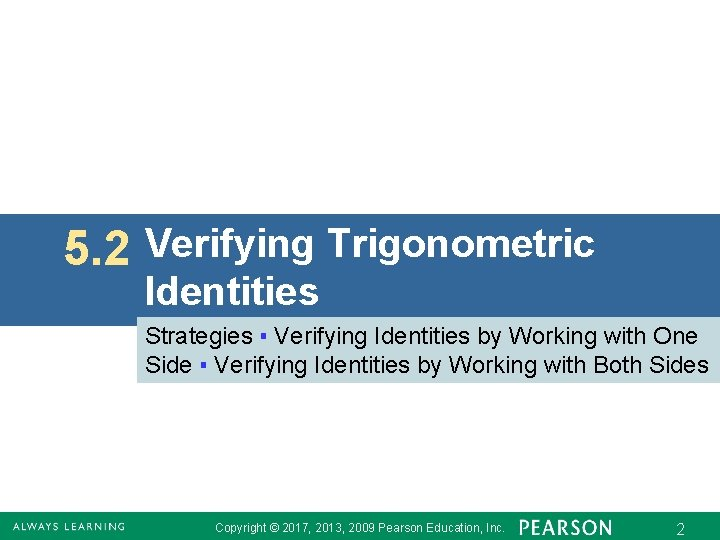5. 2 Verifying Trigonometric Identities Strategies ▪ Verifying Identities by Working with One Side ▪ Verifying Identities by Working with Both Sides Copyright © 2017, 2013, 2009 Pearson Education, Inc. 2Hints for Verifying Identities § Learn the fundamental identities. Whenever you see either side of a fundamental identity, the other side should come to mind. Also, be aware of equivalent forms of the fundamental identities. § Try to rewrite the more complicated side of the equation so that it is identical to the simpler side. Copyright © 2017, 2013, 2009 Pearson Education, Inc. 3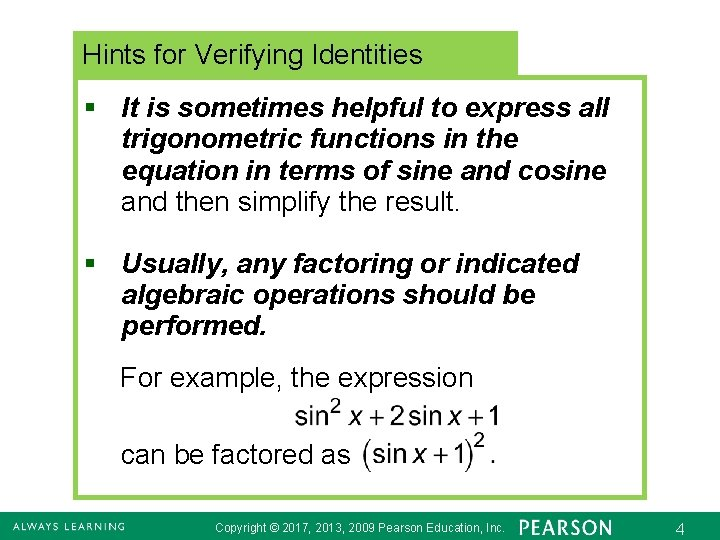Hints for Verifying Identities § It is sometimes helpful to express all trigonometric functions in the equation in terms of sine and cosine and then simplify the result. § Usually, any factoring or indicated algebraic operations should be performed. For example, the expression can be factored as Copyright © 2017, 2013, 2009 Pearson Education, Inc. 4Hints for Verifying Identities The sum or difference of two trigonometric expressions can be found in the same way as any other rational expression. For example, Copyright © 2017, 2013, 2009 Pearson Education, Inc. 5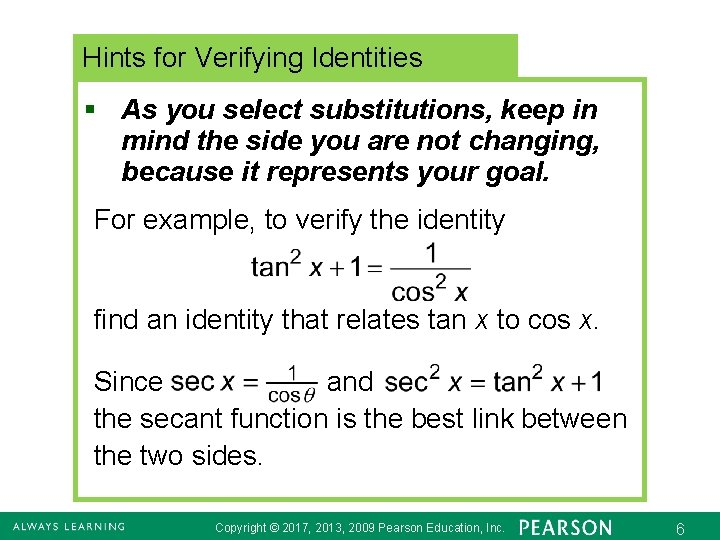Hints for Verifying Identities § As you select substitutions, keep in mind the side you are not changing, because it represents your goal. For example, to verify the identity find an identity that relates tan x to cos x. Since and the secant function is the best link between the two sides. Copyright © 2017, 2013, 2009 Pearson Education, Inc. 6Hints for Verifying Identities § If an expression contains 1 + sin x, multiplying both numerator and denominator by 1 – sin x would give 1 – sin 2 x, which could be replaced with cos 2 x. Similar procedures apply for 1 – sin x, 1 + cos x, and 1 – cos x. Copyright © 2017, 2013, 2009 Pearson Education, Inc. 7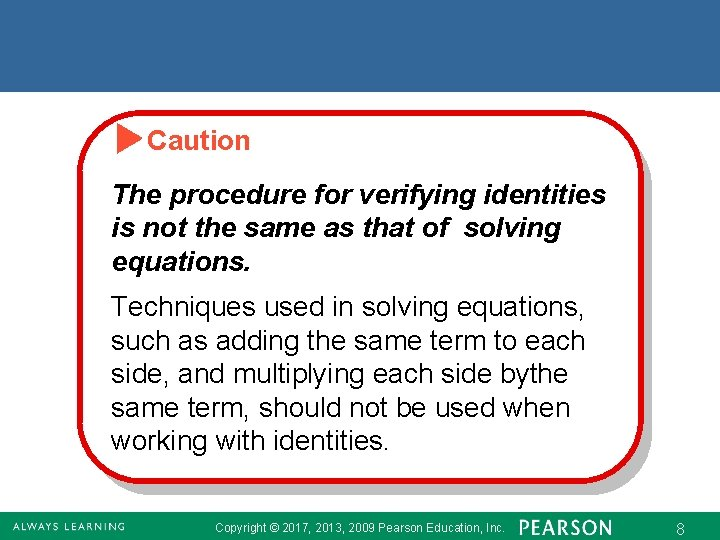Caution The procedure for verifying identities is not the same as that of solving equations. Techniques used in solving equations, such as adding the same term to each side, and multiplying each side bythe same term, should not be used when working with identities. Copyright © 2017, 2013, 2009 Pearson Education, Inc. 8Verifying Identities by Working with One Side To avoid the temptation to use algebraic properties of equations to verify identities, one strategy is to work with only one side and rewrite it to match the other side. Copyright © 2017, 2013, 2009 Pearson Education, Inc. 9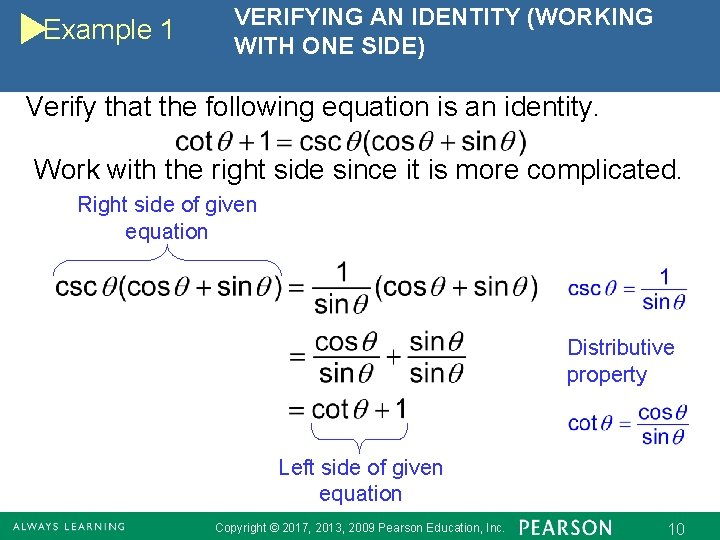Example 1 VERIFYING AN IDENTITY (WORKING WITH ONE SIDE) Verify that the following equation is an identity. Work with the right side since it is more complicated. Right side of given equation Distributive property Left side of given equation Copyright © 2017, 2013, 2009 Pearson Education, Inc. 10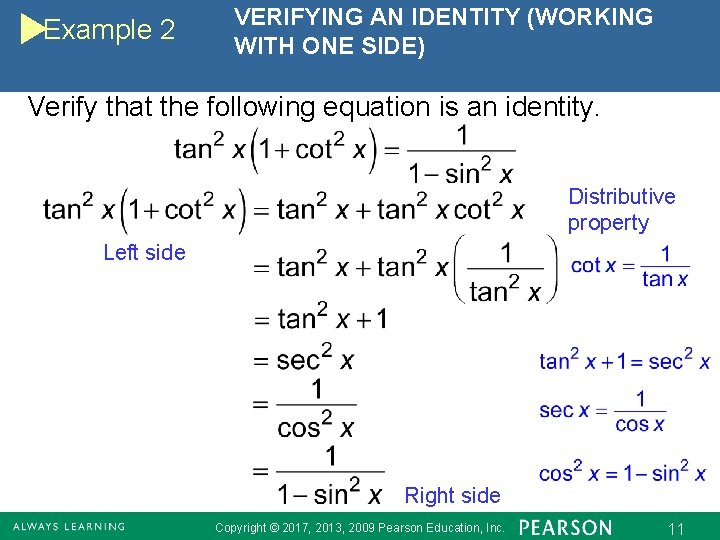Example 2 VERIFYING AN IDENTITY (WORKING WITH ONE SIDE) Verify that the following equation is an identity. Distributive property Left side Right side Copyright © 2017, 2013, 2009 Pearson Education, Inc. 11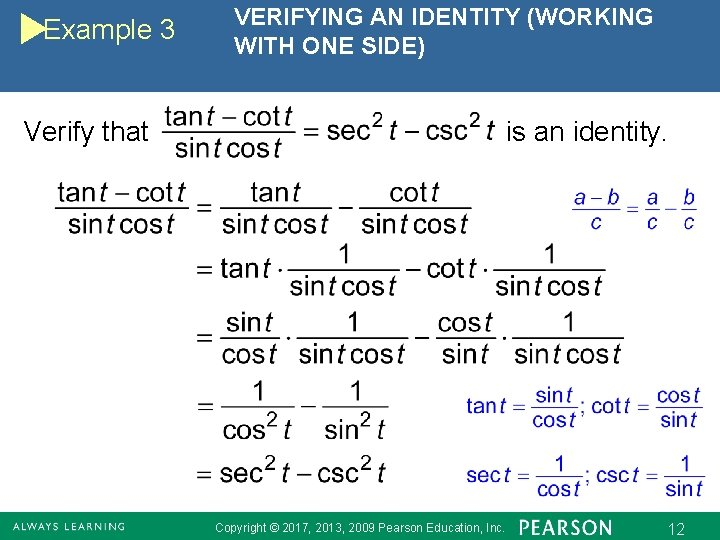Example 3 VERIFYING AN IDENTITY (WORKING WITH ONE SIDE) Verify that is an identity. Copyright © 2017, 2013, 2009 Pearson Education, Inc. 12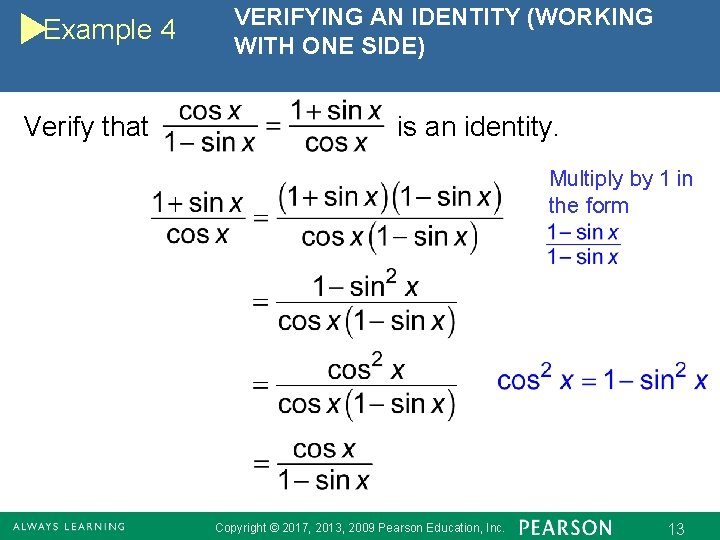Example 4 Verify that VERIFYING AN IDENTITY (WORKING WITH ONE SIDE) is an identity. Multiply by 1 in the form Copyright © 2017, 2013, 2009 Pearson Education, Inc. 13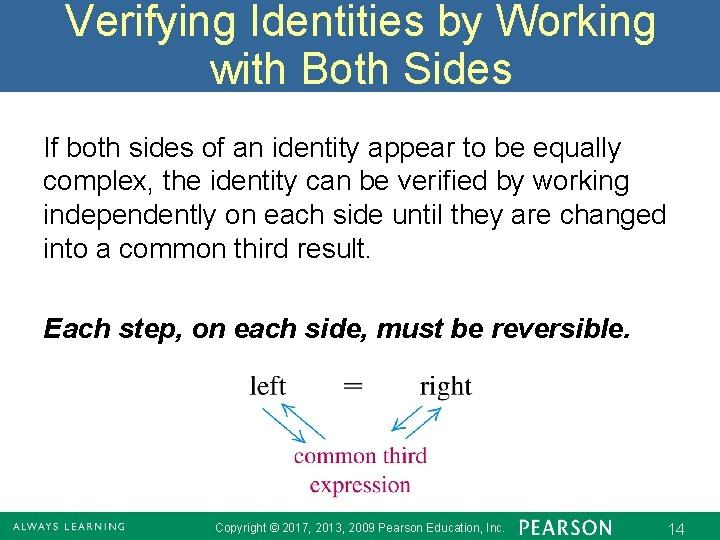Verifying Identities by Working with Both Sides If both sides of an identity appear to be equally complex, the identity can be verified by working independently on each side until they are changed into a common third result. Each step, on each side, must be reversible. Copyright © 2017, 2013, 2009 Pearson Education, Inc. 14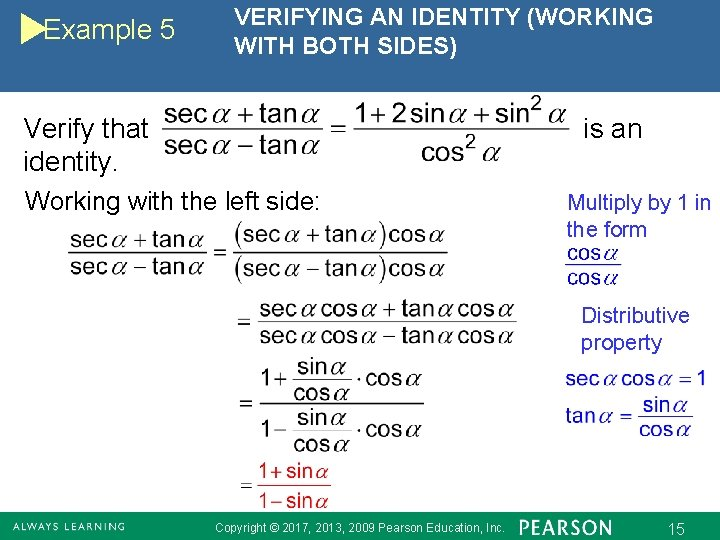Example 5 VERIFYING AN IDENTITY (WORKING WITH BOTH SIDES) Verify that identity. is an Working with the left side: Multiply by 1 in the form Distributive property Copyright © 2017, 2013, 2009 Pearson Education, Inc. 15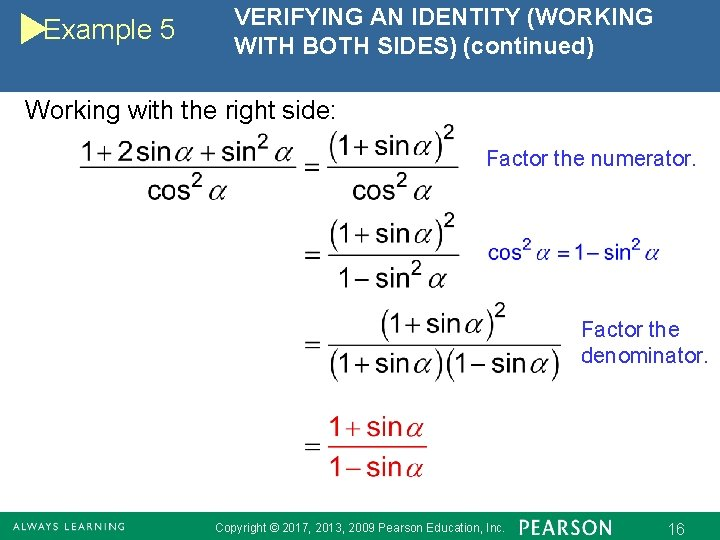Example 5 VERIFYING AN IDENTITY (WORKING WITH BOTH SIDES) (continued) Working with the right side: Factor the numerator. Factor the denominator. Copyright © 2017, 2013, 2009 Pearson Education, Inc. 16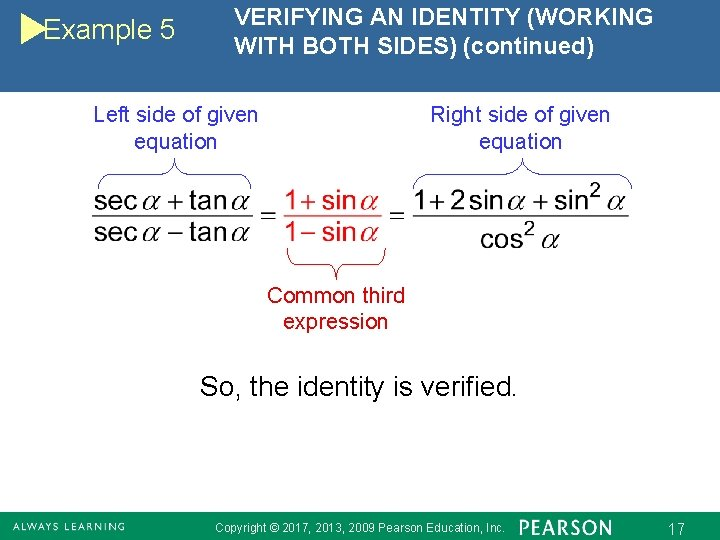Example 5 VERIFYING AN IDENTITY (WORKING WITH BOTH SIDES) (continued) Left side of given equation Right side of given equation Common third expression So, the identity is verified. Copyright © 2017, 2013, 2009 Pearson Education, Inc. 17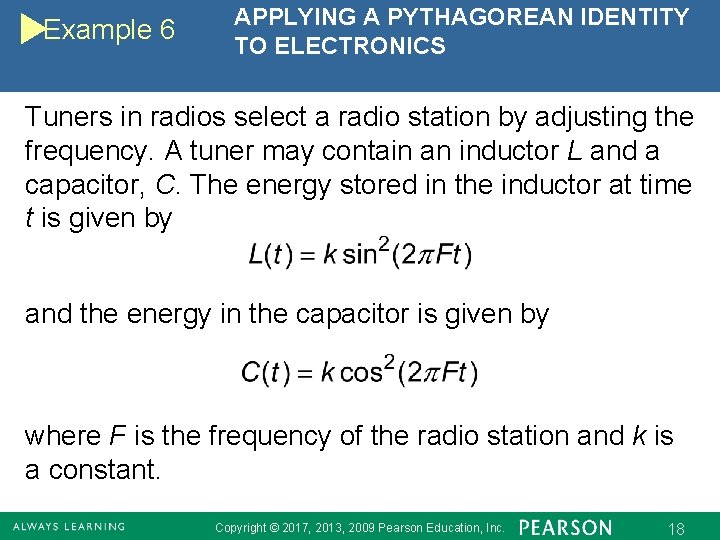Example 6 APPLYING A PYTHAGOREAN IDENTITY TO ELECTRONICS Tuners in radios select a radio station by adjusting the frequency. A tuner may contain an inductor L and a capacitor, C. The energy stored in the inductor at time t is given by and the energy in the capacitor is given by where F is the frequency of the radio station and k is a constant. Copyright © 2017, 2013, 2009 Pearson Education, Inc. 18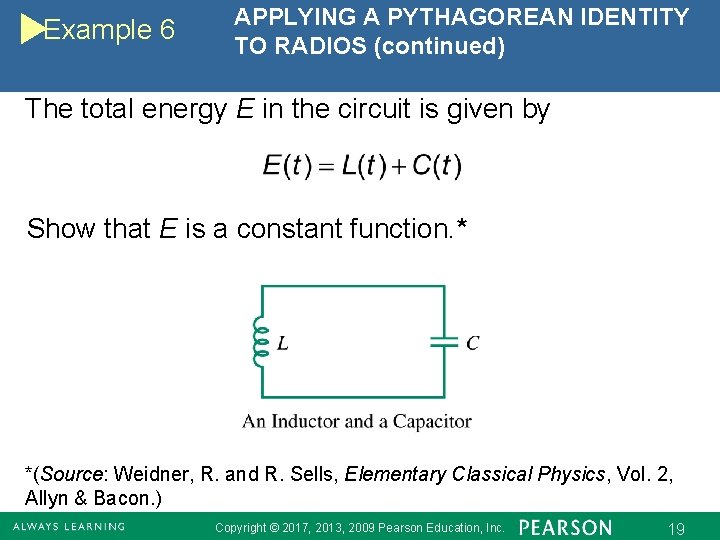Example 6 APPLYING A PYTHAGOREAN IDENTITY TO RADIOS (continued) The total energy E in the circuit is given by Show that E is a constant function. * *(Source: Weidner, R. and R. Sells, Elementary Classical Physics, Vol. 2, Allyn & Bacon. ) Copyright © 2017, 2013, 2009 Pearson Education, Inc. 19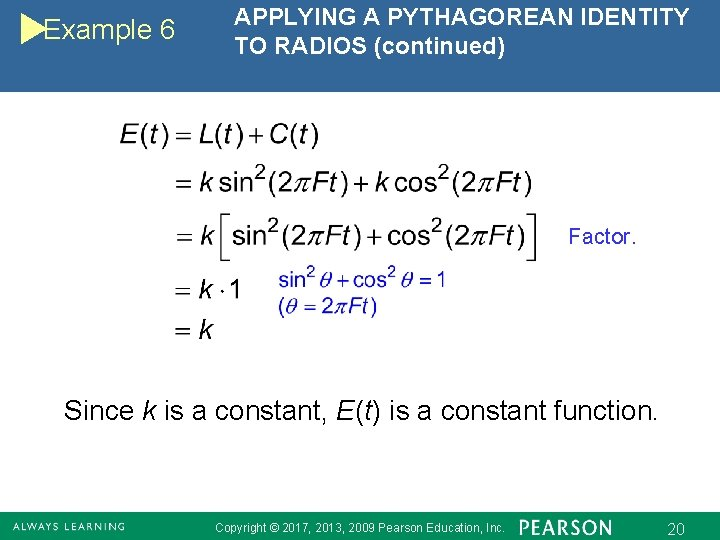Example 6 APPLYING A PYTHAGOREAN IDENTITY TO RADIOS (continued) Factor. Since k is a constant, E(t) is a constant function. Copyright © 2017, 2013, 2009 Pearson Education, Inc. 20